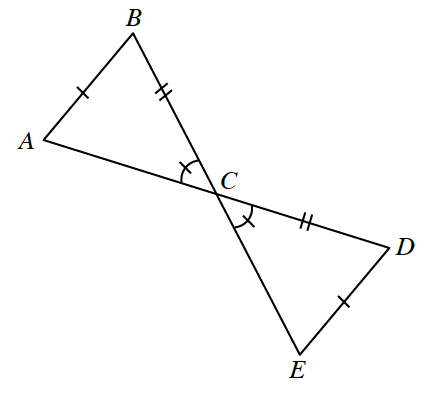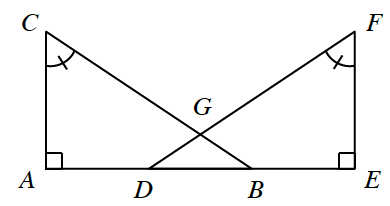### Home > INT1 > Chapter 10 > Lesson 10.2.1 > Problem10-80

10-80.

In the each of the figures below, decide if the two triangles listed are congruent. If they are congruent, create a flowchart justifying your conclusion. If they are not congruent, explain why not.

1. Is $ΔABC≅ΔEDC$ ?Review the Math Notes box in section 7.1.7

These triangles have two pairs of congruent sides, and one pair of non-included angles.
Is there an $SSA≅$ congruent conditions?

1. Is $ΔABC ≅ ΔEDF$ ?ΔDGB is isosceles.

Review the Math Notes box in section 7.1.7.
Which congruence conditions have no congruent sides in them?